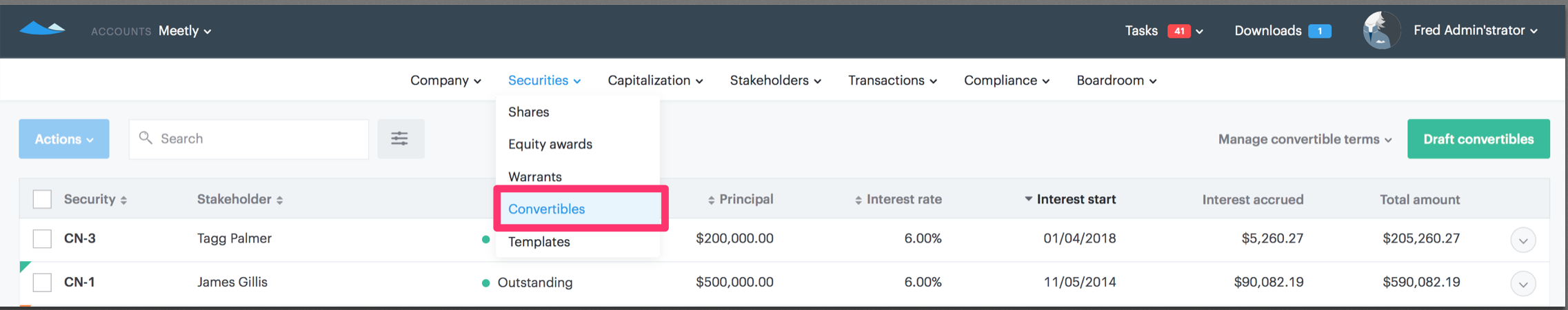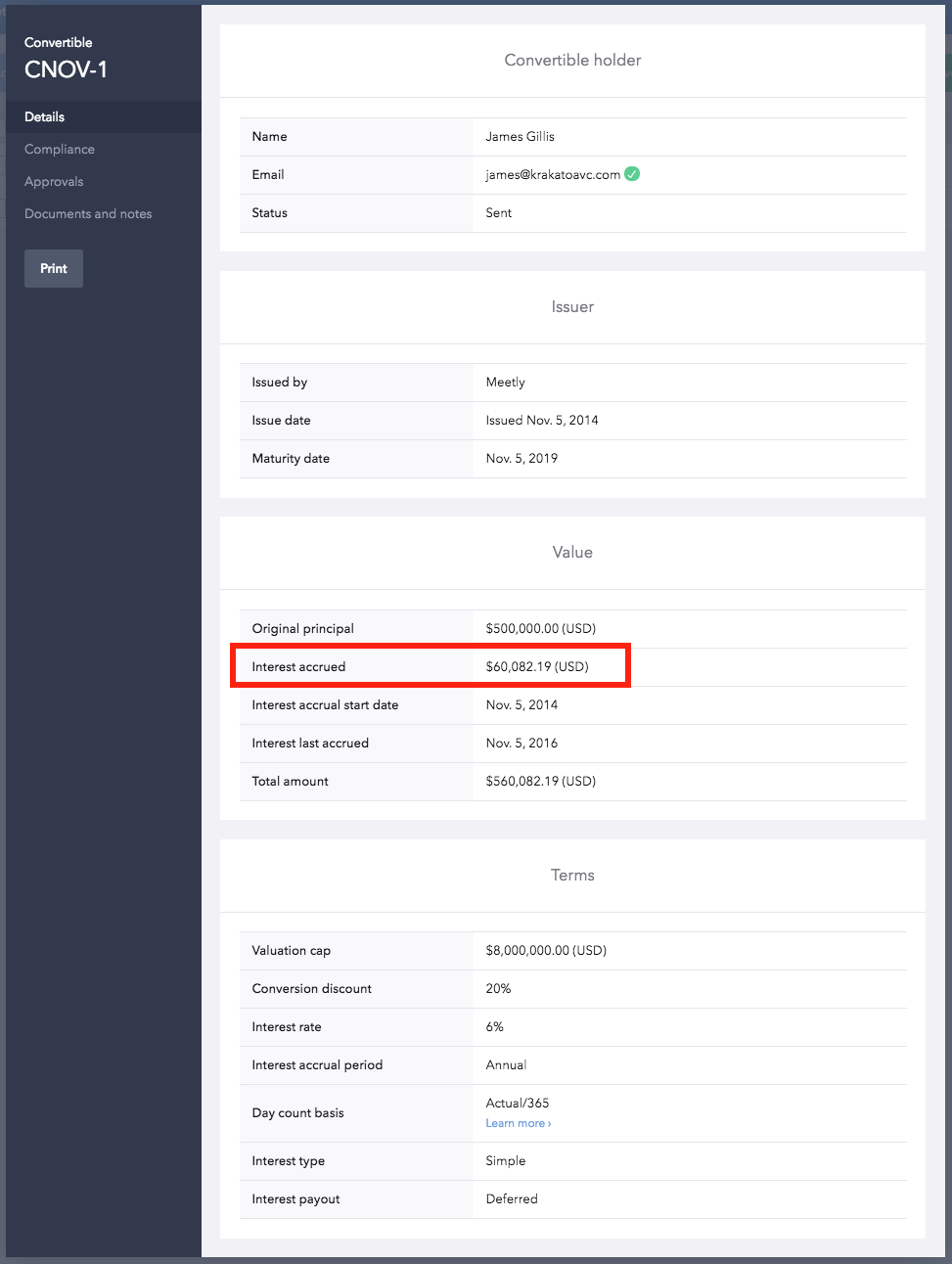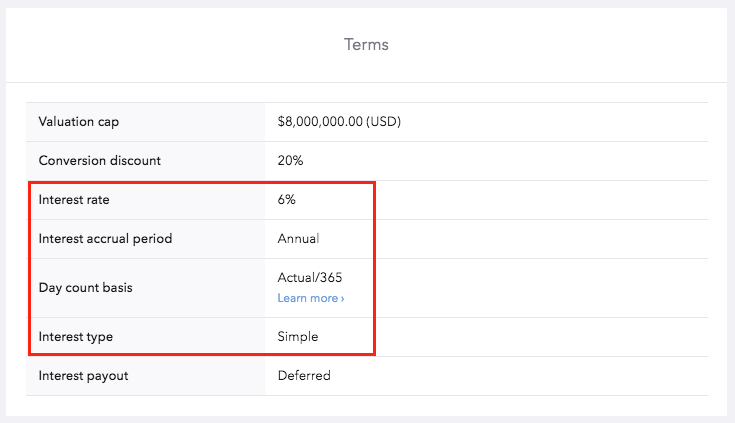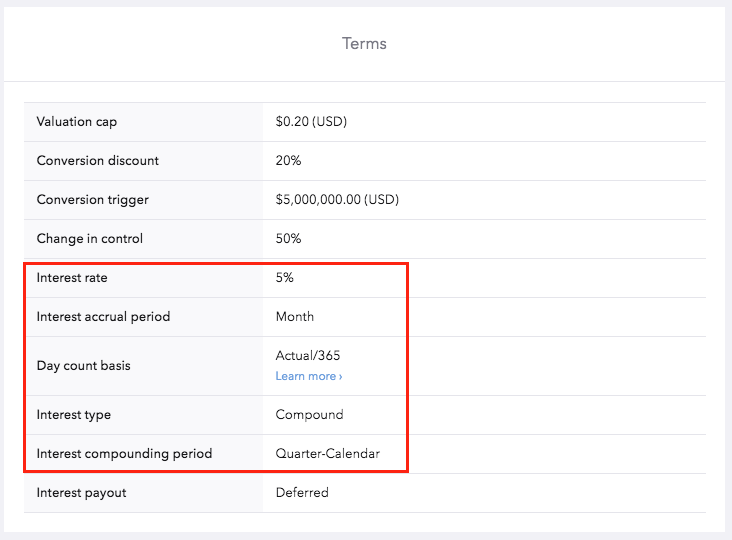# How do I find the interest payment?

#### 1. To find the interest accrued on a convertible note, click 'Convertibles' under Securities.#### 2. Select the note you want to view and you will see the interest accrued as of the interest accrual start date.Interest accrued field is based on four fields: Interest rate, Interest accrual period, day count basis and interest type.

The below calculation demonstrates the difference between the Simple Interest Method and the Compound Interest Method:

#### 1. Simple Interest Formula (Actual/365)Principal Loan Amount = \$100,000

Rate = 6%  per annum

Daily Interest = \$16.44 ((\$100,000x 0.06)/365)

In this case, Actual = 366 days (from interest start date to interest last accrued)

Annual Interest = \$6,016.44 (\$16.44 x 366/365)

Total amount = \$106,016.44

#### 2. Compound Interest Formula (Actual/365)Using compound interest formula (P*(1+i)^n) - P) :
Principal Loan Amount (P) = \$100,000
Rate = 6% per annum
Number of compounding periods per year (n)= 2
Day count basis = Actual/365
In this case, Actual = 366 days (from interest start date to interest last accrued)

Interest rate per year (i) = (0.06/2)*(366/365)

Total amount = \$106,106.93 (100,000 * (1+ (0.06/2)*(366/365))^(2)

Annual interest = 106,106.93 - 100,000= \$6,106.93.